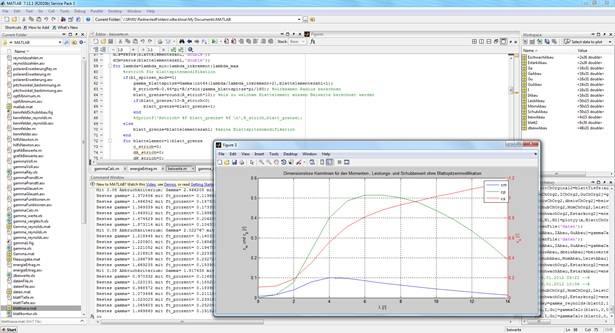Project period 01.07.2011 - 30.06.2012

To determine aerodynamic loads on wind turbine rotors characteristic maps are calculated according to the rotor blade design. At the Reiner Lemoine Institute these characteristic maps are used to calculate the actuating power of test rotors on the test bench PHiL.For this calculation the given wind speed, the measured revolution speed of the rotor and the measured pitch angles are used. The calculated rotor power then is simulated with the test bench motor using the hardware-in-the-loop principle (HiL). The calculation of characteristic maps of rotors is based on numerous parameters of blade geometry and flow theories. The program ABAUKAS should make the calculation easier and faster.

A program is developed, which can calculate characteristic maps for wind turbine rotor blades with various configurations of the blade profiles. Therefor established profiles are available through a library where further profiles easily can be added. Using the blade element theory and further modification theories the characteristic maps for torque, thrust and power coefficient can be calculated.

The necessary basics for the calculation are taken from the book "Introduction to Wind Energy".